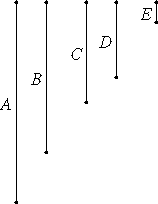# Proposition 21

Numbers relatively prime are the least of those which have the same ratio with them.

Let A and B be numbers relatively prime.

I say that A and B are the least of those which have the same ratio with them.If not, there are some numbers less than A and B in the same ratio with A and B. Let them be C and D.

VII.20

Since, then, the least numbers of those which have the same ratio measure those which have the same ratio the same number of times, the greater the greater, and the less the less, that is, the antecedent the antecedent and the consequent the consequent, therefore C measures A the same number of times that D measures B.

Let there be as many units in E as the times that C measures A. Then D also measures B according to the units in E.

VII.16

And, since C measures A according to the units in E, therefore E also measures A according to the units in C. For the same reason E also measures B according to the units in D.

VII.Def.12

Therefore E measures A and B which are relatively prime, which is impossible.

Therefore there are no numbers less than A and B which are in the same ratio with A and B. Therefore A and B are the least of those which have the same ratio with them.

Therefore, numbers relatively prime are the least of those which have the same ratio with them.

Q.E.D.

## Guide

The next proposition is the converse of this one. Together they say that a ratio a : b is reduced to lowest terms if and only if a is relatively prime to b.

Although it appears that this proposition is about pairs of numbers and their ratios, it is used in proposition VII.33 with any quantity of numbers. Stated in terms of three numbers a, b, and c, that proposition says that of all triples with the same ratio as a, b, and c, have, the triple of relatively prime numbers is least.

#### Use of this proposition

This proposition is used frequently in Books VII through IX starting with VII.24.